# Exercise 15.3 Introduction to Graphs- NCERT Solutions Class 8

## Chapter 15 Ex.15.3 Question 1

Draw the graphs for the following tables of values, with suitable scales on the axes.

(a)  Cost of apples

 Number of apples $$1$$ $$2$$ $$3$$ $$4$$ $$5$$ Cost (in Rs) $$5$$ $$10$$ $$15$$ $$20$$ $$25$$

(b) Distance travelled by a car

 Time (in hours) $$6 \,\text{am.}$$ $$7 \,\text{am.}$$ $$8 \,\text{am.}$$ $$9 \,\text{am.}$$ Distance (in km) $$40$$ $$80$$ $$120$$ $$160$$

(i) How much distance did the car cover during the period $$7.30\;\rm{a.m.}$$ to $$8\;\rm{a.m.}$$?

(ii) What was the time when the car had covered a distance of $$100 \;\rm{km}$$ since its start?

(c) Interest on deposits for a year:

 Deposit (in Rs) $$1000$$ $$2000$$ $$3000$$ $$4000$$ $$5000$$ Simple interest (in Rs) $$80$$ $$160$$ $$240$$ $$320$$ $$400$$

(i) Does the graph pass through the origin?

(ii) Use the graph to find the interest on $$\rm{Rs} \;2500$$ for a year:

(iii) To get an interest of $$\rm{Rs} \;280$$ per year, how much money should be deposited?

### Solution

Reasoning:

a) Considering ‘Number of apples’ on horizontal axis and ‘Cost (in $$\rm{Rs}$$)’ on vertical axis starting from origin i.e. ($$0,0$$) and find the value of ‘Cost’ on vertical axis according to the given value of ‘Number of apples’ on the horizontal axis value or vice versa.

b)  Considering ‘Time (in hours)’ on horizontal axis and ‘Distance (in $$\rm{km}$$)’ on vertical axis starting from origin i.e. ($$0,0$$) and find the value of ‘Distance’ on vertical axis according to the given value of ‘Time’ on the horizontal axis value orvice versa.

c) Considering ‘Deposit (in $$\rm{Rs}$$)’ on horizontal axis and ‘Simple interest (in $$\rm{Rs}$$)’ on vertical axis starting from origin i.e. ($$0,0$$) and find the value of ‘Simple interest’ on vertical axis according to the given value of ‘Deposit’ on the horizontal axis value orvice versa.

Steps:

(a) Taking a suitable scale :

For x-axis, $$2$$ unit $$= 1$$ apple and for y-axis, $$2$$ unit $$= \rm{Rs} \;5.$$

We can mark the number of apples on x-axis and the cost of apples on y-axis.

A graph of the given data is as follows.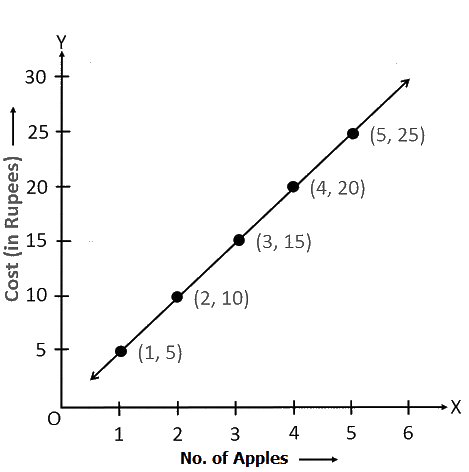(b) Taking a suitable scale

For x-axis, $$4$$ units $$= 1$$ hour and for y-axis,$$2$$ units $$= 20 \,\rm{km}$$,

We can represent the time on x-axis and the distance covered by the car on y- axis.

A graph of the given data is as follows.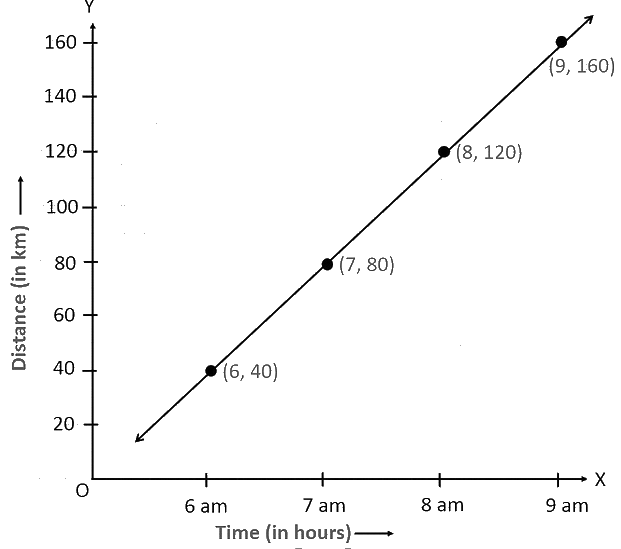(i) During the period $$7:30 \,\rm{a.m.}$$ to $$8 \rm{a.m.}$$, the car covered a distance of $$20 \,\rm{km.}$$

(ii) The car covered a distance of $$100 \,\rm{km}$$ at $$7:30 \,\rm{a.m.}$$ since its start.

(c) Taking a suitable scale,

For x-axis, $$2$$ unit = $$\rm{Rs}\, 1000$$ and for y-axis, $$2$$ unit = $$\rm{Rs}\, 50$$

We can represent the deposit on x-axis and the interest earned on that deposit on y-axis. A graph of the given data is obtained as follows.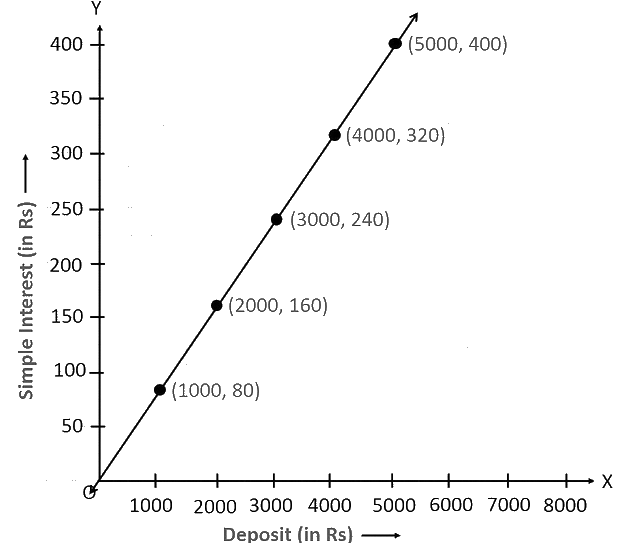From the graph, the following points can be observed.

(i) Yes. The graph passes through the origin.

(ii) The interest earned in a year on a deposit of $$\rm{Rs}\, 2500$$ is $$\rm{Rs}\, 200.$$

(iii) To get an interest of $$\rm{Rs}\,280$$ per year, $$\rm{Rs} \,3500$$ should be deposited.

## Chapter 15 Ex.15.3 Question 2

Draw a graph for the following.

(i)

 Side of square (in $$\rm{cm}$$) $$2$$ $$3$$ $$3.5$$ $$5$$ $$6$$ Perimeter (in $$\rm{cm}$$) $$8$$ $$12$$ $$14$$ $$20$$ $$24$$

Is it a linear graph?

(ii)

 Side of square (in $$\rm{cm}$$) $$2$$ $$3$$ $$4$$ $$5$$ $$6$$ Area (in $$\rm{cm}^2$$) $$4$$ $$9$$ $$16$$ $$25$$ $$36$$

Is it a linear graph?

### Solution

Reasoning:

A line graph which is a whole unbroken line is called a linear graph.

Steps:

(i) Choosing a suitable scale,

For $$x$$-axis, $$2$$ unit $$= 1\, \rm{cm}$$ and for $$y$$-axis, $$2$$ unit $$= 5\, \rm{cm}$$

We can represent the side of a square on $$x$$-axis and the perimeter of that square on $$y$$-axis. A graph of the given data is drawn as follows.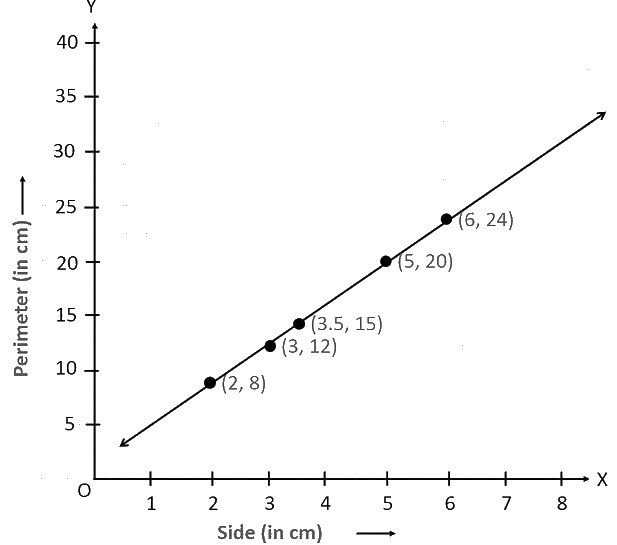It is a linear graph.

(ii) Choosing a suitable scale,

For x-axis, $$2$$ unit $$= 1 \,\rm{cm}$$ and for $$y$$-axis, $$2$$ unit $$= 5\;\rm{cm}$$

We can represent the side of a square on the x-axis and the area of that square on $$y$$-axis. A graph of the given data is as follows.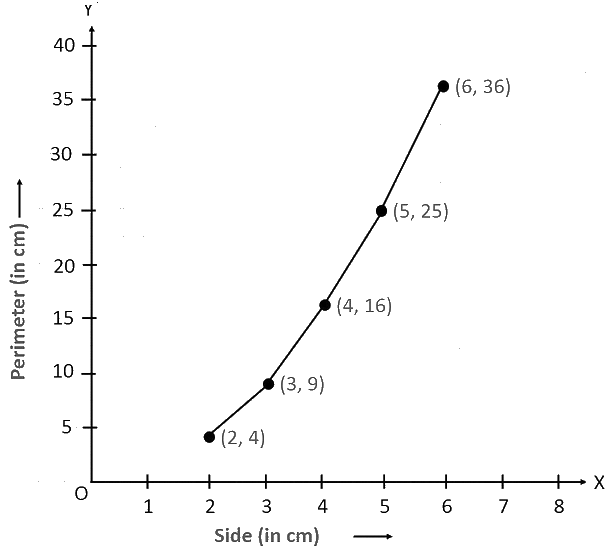It is not a linear graph.

Instant doubt clearing with Cuemath Advanced Math Program